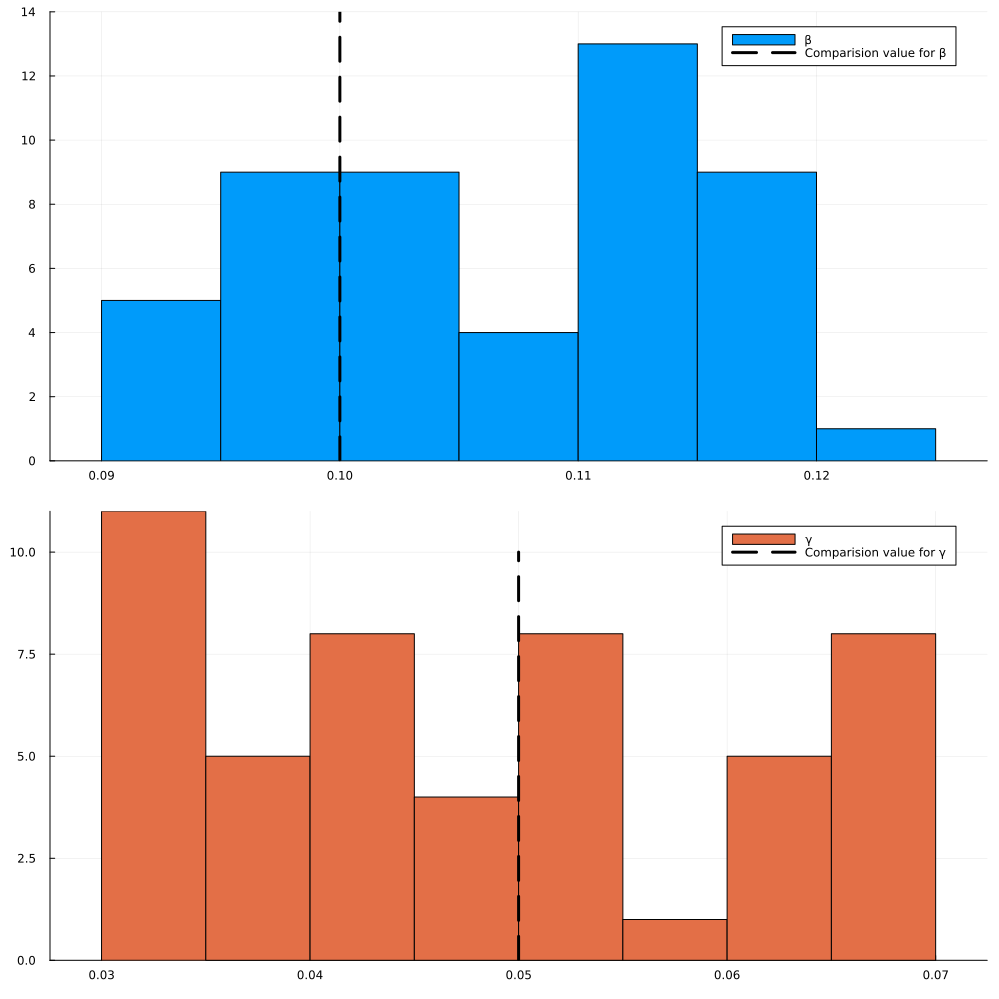# Recovery of Epidemic Parameters in Models of Infectious Disease

Here we present an epidemiology example combining ModelingToolkit with JuliaModelSimOptimizer. This example is based on the classical SIR model of epidemiology. The SIR models consists of 3 compartments: Susceptible (S), Infected (I) and Recovered (R). The contact rate (β) and the recovery rate (γ) govern the transfer of population from susceptible to infected, and infected to recovered respectively.

## Julia environment

# Packages used for this example
using JuliaSimModelOptimizer
using OrdinaryDiffEq
using ModelingToolkit
using DataFrames
using Statistics
using StatsPlots
Plots.GRBackend()

## Model setup

The model setup involves the 3 basic equations which govern the SIR model. These equations have been written in their symbolic form using the ModelingToolkit library.

iv = @variables t
states = @variables S(t) I(t) R(t)

ps = @parameters β γ

D = Differential(t)
eqs = [D(S) ~ -β*S*I,
D(I) ~ β*S*I - γ*I,
D(R) ~ γ*I]

defs = Dict(S => 0.99,
I=> 0.01,
R => 0.0,
β => 0.1,
γ => 0.05
)

@named model = ODESystem(eqs, t, states, ps; defaults = defs, checks = false)

trial_inits = [S => 0.99,
I=> 0.01,
R => 0.0,
]

trial_ps = [β => 0.1,
γ => 0.05
]
trial_tspan = (0.0, 100.)

trial_n = 10
trial_saveat = range(trial_tspan, trial_tspan, length = trial_n)
trial_prob = ODEProblem(model, trial_inits, trial_tspan, trial_ps, saveat = trial_saveat)
trial_sol = solve(trial_prob, Tsit5(), reltol=1e-8, abstol=1e-8)
retcode: Success
Interpolation: 1st order linear
t: 10-element Vector{Float64}:
0.0
11.11111111111111
22.22222222222222
33.333333333333336
44.44444444444444
55.55555555555556
66.66666666666667
77.77777777777777
88.88888888888889
100.0
u: 10-element Vector{Vector{Float64}}:
[0.99, 0.01, 0.0]
[0.9755251109170952, 0.017110368147264958, 0.007364520935639738]
[0.9514984480410545, 0.02866810835254597, 0.01983344360639943]
[0.9132105003651881, 0.04642023489052607, 0.0403692647442857]
[0.8559458639132691, 0.07130523057758646, 0.07274890550914433]
[0.777789195790239, 0.10158609814023152, 0.12062470606952942]
[0.6830751115365429, 0.1313748283327412, 0.18555006013071582]
[0.5827966947253064, 0.15227003551005383, 0.2649332697646397]
[0.48970339203160695, 0.1583440772109222, 0.3519525307574709]
[0.4121864470739073, 0.149698975621419, 0.43811457730467374]

## Creating the inverse problem

After generating the solution above, we can convert it into a data frame and generate an Experiment object. This Experiment object can then be fed into the InverseProblem interface. An inverse problem is a collection of trials which define a multi-simulation optimization problem. The solution to an inverse problem are the "good parameters" which make the simulations simultaniously fit their respective data sufficiently well. Next to the model and the trials, the user needs to specify a search space. This is to constrain the optimiser in which values to consider as potential values for the parameter combinations.

experiment_data = DataFrame(trial_sol)

experiment = Experiment(experiment_data, model;
u0 = trial_inits,
params = trial_ps,
tspan = trial_tspan
)

prob = InverseProblem(experiment, model, [β => (0.09, 0.12), γ => (0.03, 0.07)])
InverseProblem for model with one experiment optimizing 2 parameters.


## Generating and visualizing the optimized parameters

Once the inverse problem is defined, we can generate a virtual population of all the parameters and visualize the results! In this example, we are simultaneously optimizing for 2 parameters: the contact rate (β) and the recovery rate (γ).

ps = parametric_uq(prob, StochGlobalOpt(maxiters=10), sample_size = 50)

plot(ps, layout=(2,1), bins = 10, show_comparision_value = true, max_freq = [14, 10], legend = :best, size = (1000,1000))As we see above, both the contact rate and the recovery rate match the underlying true parameters very well!